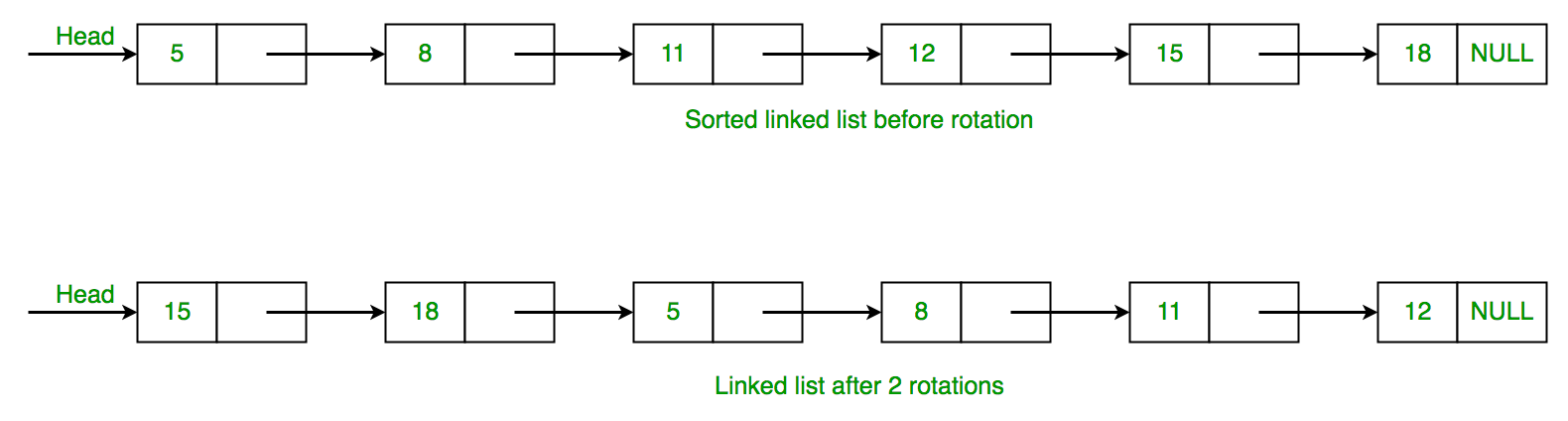Related Articles
Count rotations in sorted and rotated linked list
• Difficulty Level : Easy
• Last Updated : 31 Dec, 2020

Given a linked list of n nodes which is first sorted, then rotated by k elements. Find the value of k.The idea is to traverse singly linked list to check condition whether current node value is greater than value of next node. If the given condition is true, then break the loop. Otherwise increase the counter variable and increase the node by node->next. Below is the implementation of this approach.

## C++

 `// Program for count number of rotations in``// sorted linked list.``#include ``using` `namespace` `std;``/* Linked list node */``struct` `Node {``    ``int` `data;``    ``struct` `Node* next;``};`` ` `// Function that count number of``// rotation in singly linked list.``int` `countRotation(``struct` `Node* head)``{``    ``// declare count variable and assign it 1.``    ``int` `count = 0;`` ` `    ``// declare a min variable and assign to``    ``// data of head node.``    ``int` `min = head->data;`` ` `    ``// check that while head not equal to NULL.``    ``while` `(head != NULL) {`` ` `        ``// if min value is greater then head->data``        ``// then it breaks the while loop and``        ``// return the value of count.``        ``if` `(min > head->data)``            ``break``;`` ` `        ``count++;`` ` `        ``// head assign the next value of head.``        ``head = head->next;``    ``}``    ``return` `count;``}`` ` `// Function to push element in linked list.``void` `push(``struct` `Node** head, ``int` `data)``{``    ``// Allocate dynamic memory for newNode.``    ``struct` `Node* newNode = ``new` `Node;`` ` `    ``// Assign the data into newNode.``    ``newNode->data = data;`` ` `    ``// newNode->next assign the address of``    ``// head node.``    ``newNode->next = (*head);`` ` `    ``// newNode become the headNode.``    ``(*head) = newNode;``}`` ` `// Display linked list.``void` `printList(``struct` `Node* node)``{``    ``while` `(node != NULL) {``        ``printf``(``"%d "``, node->data);``        ``node = node->next;``    ``}``}`` ` `// Driver functions``int` `main()``{``    ``// Create a node and initialize with NULL``    ``struct` `Node* head = NULL;`` ` `    ``// push() insert node in linked list.``    ``// 15 -> 18 -> 5 -> 8 -> 11 -> 12``    ``push(&head, 12);``    ``push(&head, 11);``    ``push(&head, 8);``    ``push(&head, 5);``    ``push(&head, 18);``    ``push(&head, 15);`` ` `    ``printList(head);``    ``cout << endl;``    ``cout << ``"Linked list rotated elements: "``;`` ` `    ``// Function call countRotation()``    ``cout << countRotation(head) << endl;`` ` `    ``return` `0;``}`

## Python3

 `# Program for count number of rotations in``# sorted linked list.`` ` `# Linked list node ``class` `Node:``     ` `    ``def` `__init__(``self``, data):``         ` `        ``self``.data ``=` `data``        ``self``.``next` `=` `None`` ` `# Function that count number of``# rotation in singly linked list.``def` `countRotation(head):`` ` `    ``# Declare count variable and assign it 1.``    ``count ``=` `0``  ` `    ``# Declare a min variable and assign to``    ``# data of head node.``    ``min` `=` `head.data``  ` `    ``# Check that while head not equal to None.``    ``while` `(head !``=` `None``):``  ` `        ``# If min value is greater then head->data``        ``# then it breaks the while loop and``        ``# return the value of count.``        ``if` `(``min` `> head.data):``            ``break``  ` `        ``count ``+``=` `1``  ` `        ``# head assign the next value of head.``        ``head ``=` `head.``next``     ` `    ``return` `count`` ` `# Function to push element in linked list.``def` `push(head, data):`` ` `    ``# Allocate dynamic memory for newNode.``    ``newNode ``=` `Node(data)``  ` `    ``# Assign the data into newNode.``    ``newNode.data ``=` `data``  ` `    ``# newNode->next assign the address of``    ``# head node.``    ``newNode.``next` `=` `(head)``  ` `    ``# newNode become the headNode.``    ``(head) ``=` `newNode``    ``return` `head`` ` `# Display linked list.``def` `printList(node):`` ` `    ``while` `(node !``=` `None``):``        ``print``(node.data, end ``=` `' '``)``        ``node ``=` `node.``next``     ` `# Driver code``if` `__name__``=``=``'__main__'``:``     ` `    ``# Create a node and initialize with None``    ``head ``=` `None``  ` `    ``# push() insert node in linked list.``    ``# 15 -> 18 -> 5 -> 8 -> 11 -> 12``    ``head ``=` `push(head, ``12``)``    ``head ``=` `push(head, ``11``)``    ``head ``=` `push(head, ``8``)``    ``head ``=` `push(head, ``5``)``    ``head ``=` `push(head, ``18``)``    ``head ``=` `push(head, ``15``)``  ` `    ``printList(head);``    ``print``()``    ``print``(``"Linked list rotated elements: "``, ``          ``end ``=` `'')``  ` `    ``# Function call countRotation()``    ``print``(countRotation(head))`` ` `# This code is contributed by rutvik_56`
Output
```15 18 5 8 11 12
Linked list rotated elements: 2```

This article is contributed by Dharmendra Kumar. If you like GeeksforGeeks and would like to contribute, you can also write an article using contribute.geeksforgeeks.org or mail your article to contribute@geeksforgeeks.org. See your article appearing on the GeeksforGeeks main page and help other Geeks.
Please write comments if you find anything incorrect, or you want to share more information about the topic discussed above.

Attention reader! Don’t stop learning now. Get hold of all the important DSA concepts with the DSA Self Paced Course at a student-friendly price and become industry ready.

My Personal Notes arrow_drop_up